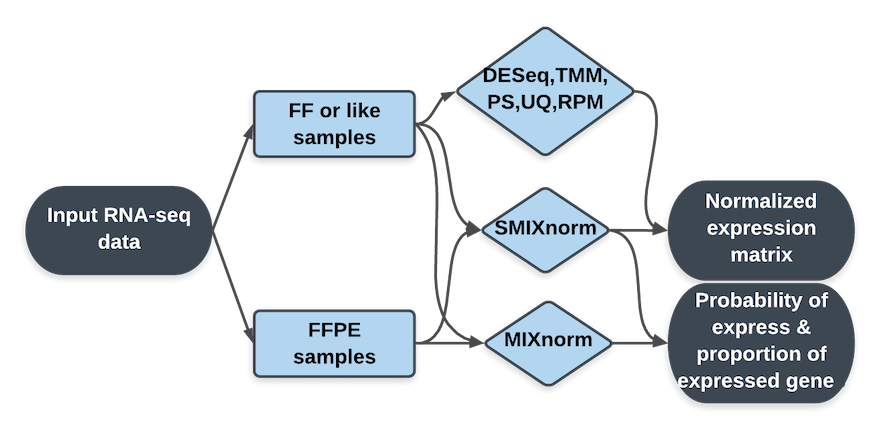## Architect of Our ToolsAll methods take raw count matrix as input and reture the normalized expression matrix as a downloadable csv file. Offline versions of MIXnorm and SMIXnom are available from the Download page. The probability of being expressed for each gene and the proportion of expressed genes are also returned from the offline version.

## Main Functions

### MIXnorm

MIXnorm is based on a two-component mixture model, which intends to capture major characteristics of the FFPE RNA-seq data accurately. MIXnorm takes the raw count data as input and output the normalized expression matrix in log scale.

### SMIXnorm

SMIXnorm is a simplified version of MIXnorm. We recommend using SMIXnorm for FFPE RNA-seq data normalization for faster computation when the number of samples is larger than 25.

### DESeq

DESeq normalizes the raw data by using (estimated) scaling factors and is implemented in R package DESeq. The scaling factors are calculated by the median ratio of gene counts to its geometric mean across samples.

### The trimmed mean of M-values normalization (TMM)

TMM is implemented in edgeR package. The scaling factor is estimated by the weighted mean of M values after trimmed away the extreme M values.

### PoissonSeq

PoissonSeq is implemented from PoissonSeq package. PoissonSeq models raw RNA-seq count data by a Poisson log-linear model. The normalization is performed implicitly.

### The reads per million normalization (RPM)

The RPM is perhaps the most straight forward normalization method. RPM estimates the scaling factor by the total number of reads for each sample.

### The upper-quartile normalization (UQ)

The UQ normalization estimates the scaling factor by the upper quartile of read counts across all genes for each sample.